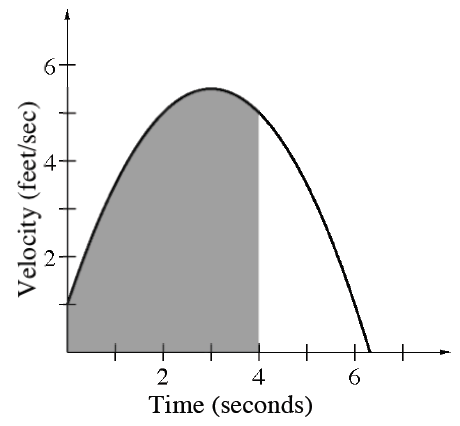### Home > APCALC > Chapter 4 > Lesson 4.2.3 > Problem4-73

4-73.

The graph at right shows the velocity of an object over time defined by the function $v\left(t\right) = −0.5t^{2} + 3t + 1$.

1. Use your graphing calculator to evaluate $\int _ { 0 } ^ { 4 } ( - 0.5 t ^ { 2 } + 3 t + 1 ) d t$.

$17\frac{1}{3}$

2. What does the result in part (a) calculate?

You just calculated the area under a velocity graph.

3. What does $\frac { d } { d t } ( v ( t ) )$ represent?

Translation 1: What is the derivative of a velocity function?
Translation 2: What is the slope function of a velocity function?

Translation 3: If velocity is the derivative of position, then what is the derivative of velocity?

4. What will the units be in part (c)?

$\text{Evaluate and simplify: }\frac{v(t)}{t}, \text{ where }v(t)=\frac{\text{feet}}{\text{seconds}}\text{ and }t=\text{ seconds.}$